### IMO Shortlist 2012 problem G3

Kvaliteta:
Avg: 4,0
Težina:
Avg: 7,0
In an acute triangle$ABC$ the points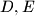$D,E$ and$F$ are the feet of the altitudes through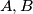$A,B$ and$C$ respectively. The incenters of the triangles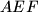$AEF$ and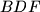$BDF$ are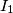$I_1$ and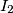$I_2$ respectively; the circumcenters of the triangles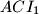$ACI_1$ and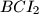$BCI_2$ are$O_1$ and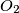$O_2$ respectively. Prove that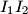$I_1I_2$ and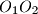$O_1O_2$ are parallel.
Izvor: Međunarodna matematička olimpijada, shortlist 2012